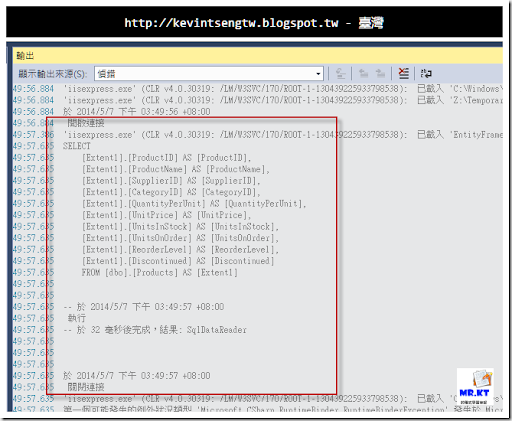## 2014年4月25日 星期五

### 基本操作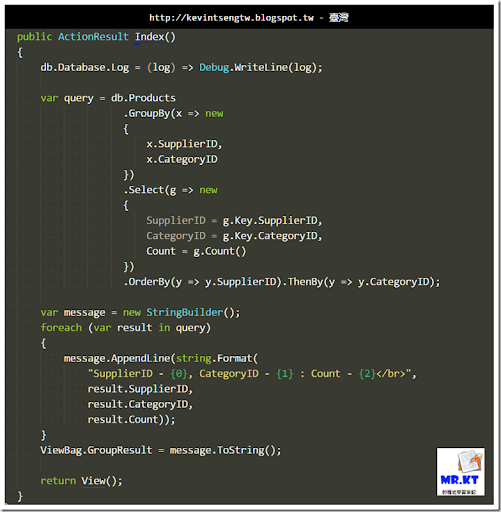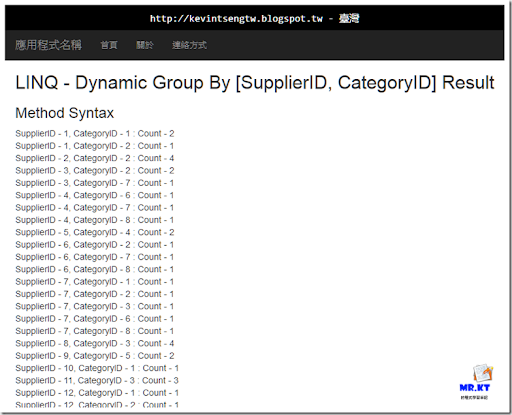T-SQL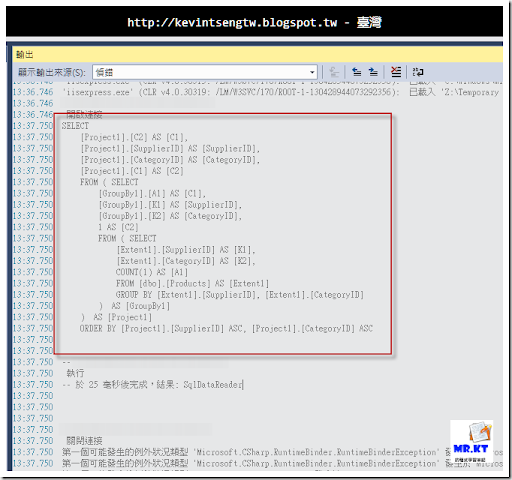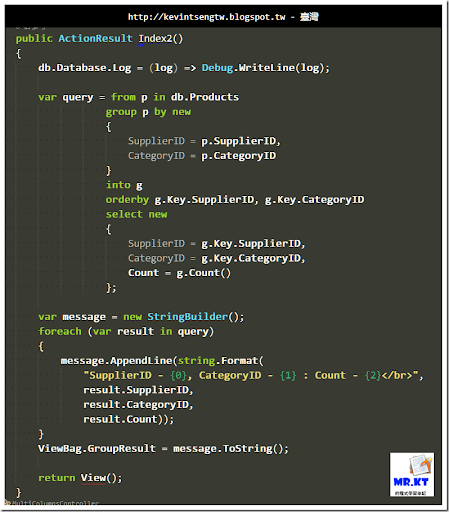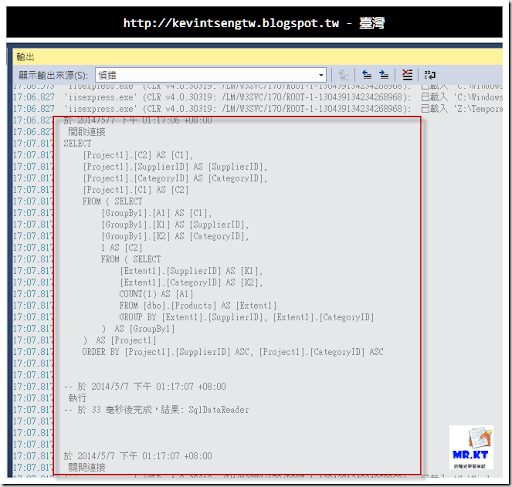### 使用 DynamicQuery（Dynamic Expression API）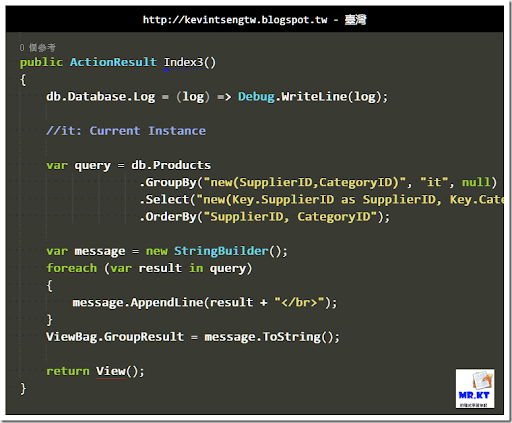`public ActionResult Index3()`
`{`
`    db.Database.Log = (log) => Debug.WriteLine(log);`
` `
`    //it: Current Instance`
` `
`    var query = db.Products`
`                  .GroupBy("new(SupplierID,CategoryID)", "it", null)`
`                  .Select("new(Key.SupplierID as SupplierID, Key.CategoryID as CategoryID, Count() as Count)")`
`                  .OrderBy("SupplierID, CategoryID");`
` `
`    var message = new StringBuilder();`
`    foreach (var result in query)`
`    {`
`        message.AppendLine(result + "</br>");`
`    }`
`    ViewBag.GroupResult = message.ToString();`
` `
`    return View();`
`}`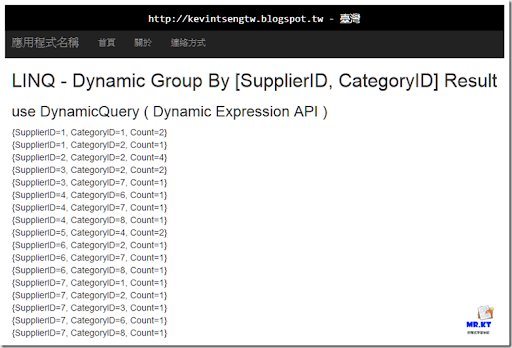T-SQL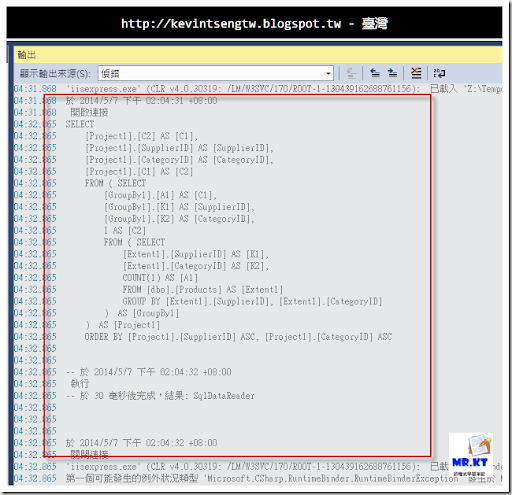`public ActionResult Index3()`
`{`
`    db.Database.Log = (log) => Debug.WriteLine(log);`
` `
`    //it: Current Instance`
` `
`    var query = db.Products`
`                  .GroupBy("new(SupplierID,CategoryID)", "it", null)`
`                  .Select("new(Key.SupplierID as SupplierID, Key.CategoryID as CategoryID, Count() as Count)")`
`                  .OrderBy("SupplierID, CategoryID");`
` `
`    var message = new StringBuilder();`
`    foreach (var result in query)`
`    {`
`        message.AppendLine(result + "</br>");`
`    }`
`    ViewBag.GroupResult = message.ToString();`
` `
`    return View();`
`}`

### 使用 GroupByMany 方法

twMVC 的夥伴「Bruce Chen 陳傳興」對於 LINQ 動態多欄位 Group 的處理提供了兩篇文章參考，

Playing with Linq grouping: GroupByMany ? – Mitsu’s blog- Site Home - MSDN Blogs

Linq GroupByMany dynamically – Mitsu’s blog - Site Home - MSDN Blogs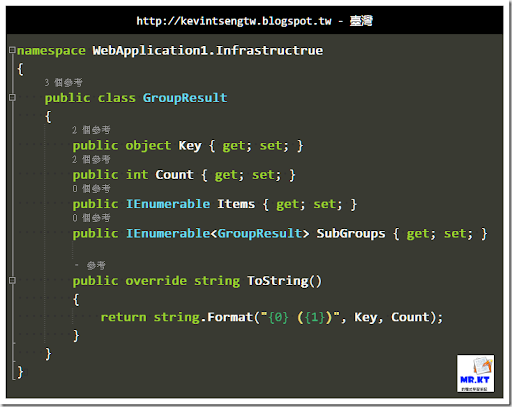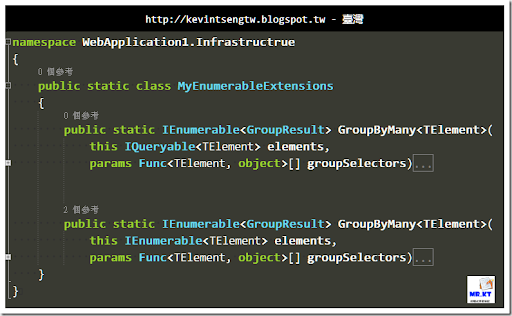`public static class MyEnumerableExtensions`
`{`
`    public static IEnumerable<GroupResult> GroupByMany<TElement>(`
`        this IQueryable<TElement> elements,`
`        params Func<TElement, object>[] groupSelectors)`
`    {`
`        if (groupSelectors.Length > 0)`
`        {`
`            var selector = groupSelectors.First();`
` `
`            //reduce the list recursively until zero`
`            var nextSelectors = groupSelectors.Skip(1).ToArray();`
`            var result = elements`
`                .GroupBy(selector)`
`                .Select(`
`                    g => new GroupResult`
`                    {`
`                        Key = g.Key,`
`                        Count = g.Count(),`
`                        Items = g,`
`                        SubGroups = g.GroupByMany(nextSelectors)`
`                    });`
` `
`            return result;`
`        }`
`        else`
`        {`
`            return null;`
`        }`
`    }`
` `
` `
`    public static IEnumerable<GroupResult> GroupByMany<TElement>(`
`        this IEnumerable<TElement> elements,`
`        params Func<TElement, object>[] groupSelectors)`
`    {`
`        if (groupSelectors.Length > 0)`
`        {`
`            var selector = groupSelectors.First();`
` `
`            //reduce the list recursively until zero`
`            var nextSelectors = groupSelectors.Skip(1).ToArray();`
`            var result = elements`
`                .GroupBy(selector)`
`                .Select(`
`                    g => new GroupResult`
`                    {`
`                        Key = g.Key,`
`                        Count = g.Count(),`
`                        Items = g,`
`                        SubGroups = g.GroupByMany(nextSelectors)`
`                    });`
` `
`            return result;`
`        }`
`        else`
`        {`
`            return null;`
`        }`
`    }`
`}`

Controller 內的程式做法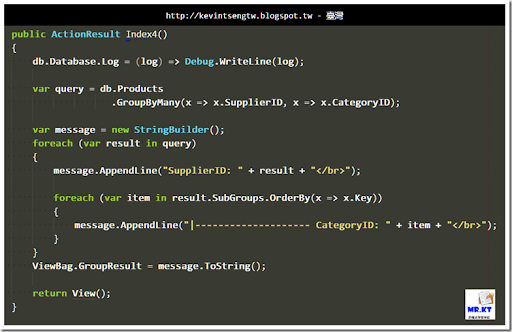View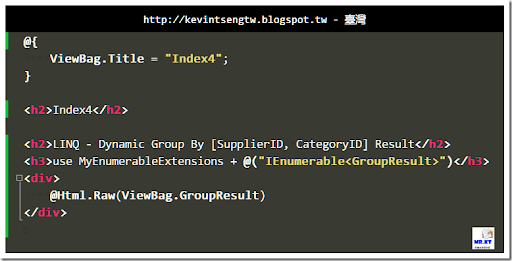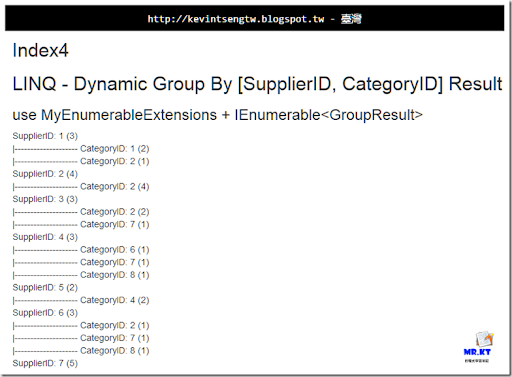T-SQL